# Consider the reaction. 2 A(g) + B(g) K, = 2.02 x 10-6 at 500 K If...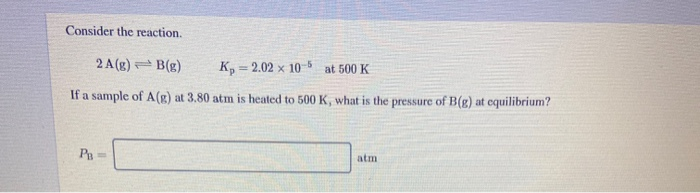Consider the reaction. 2 A(g) + B(g) K, = 2.02 x 10-6 at 500 K If a sample of A(R) at 3.80 atm is heated to 500 K, what is the pressure of B(g) at equilibrium? atm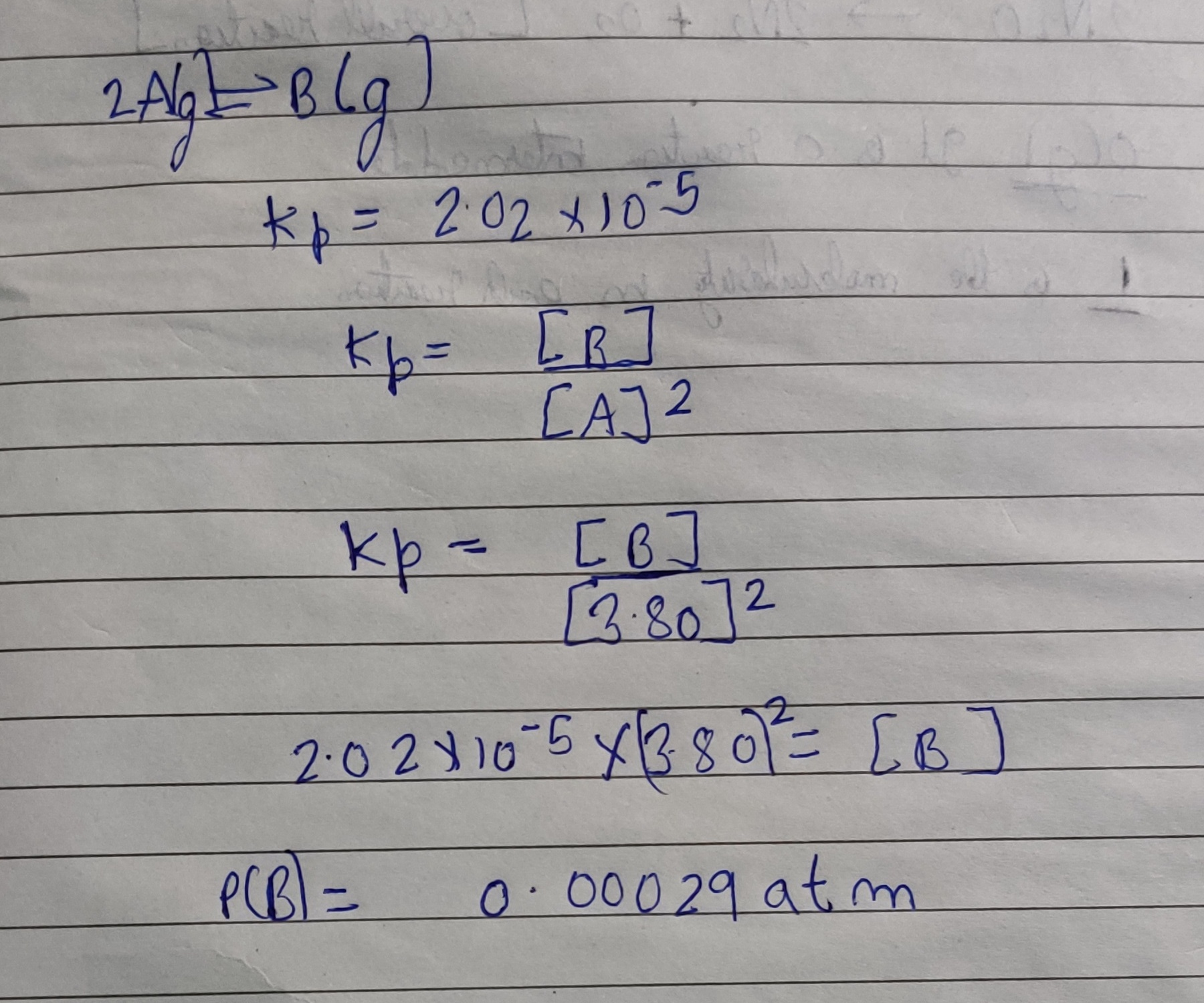#### Earn Coin

Coins can be redeemed for fabulous gifts.

Similar Homework Help Questions
• ### Consider the reaction. 2 A(g) – B(g) K = 5.90 x 10-5 at 500 K If...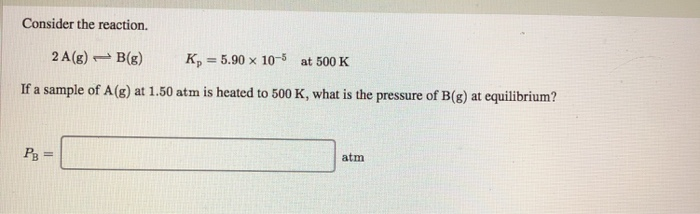Consider the reaction. 2 A(g) – B(g) K = 5.90 x 10-5 at 500 K If a sample of A(g) at 1.50 atm is heated to 500 K, what is the pressure of B(8) at equilibrium? P₂ = atm

• ### Consider the reaction. A(aq)↽−−⇀2B(aq)?c=2.02×10−6 at 500 K If a 4.20 M sample of A is heated...

Consider the reaction. A(aq)↽−−⇀2B(aq)?c=2.02×10−6 at 500 K If a 4.20 M sample of A is heated to 500 K, what is the concentration of B at equilibrium?

• ### Consider the reaction. A(aq) = 3 B(aq) Kc = 9.91 x 10-6 at 500 K If a 3.80 M sample of A is heated to 500 K, what i...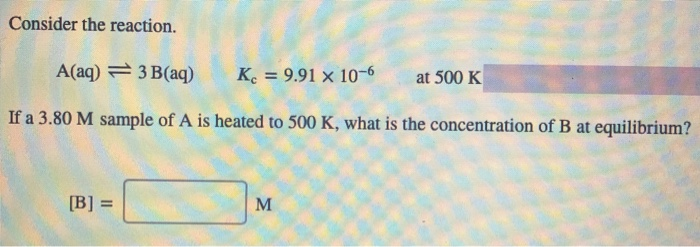Consider the reaction. A(aq) = 3 B(aq) Kc = 9.91 x 10-6 at 500 K If a 3.80 M sample of A is heated to 500 K, what is the concentration of B at equilibrium? [B] =

• ### Consider the reaction. A(aq) = 2 B(aq) K = 3.52 x 10-6 at 500 K If...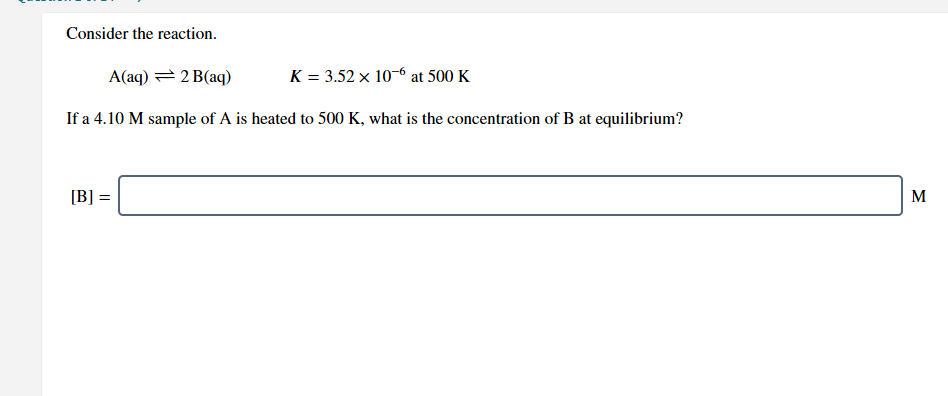Consider the reaction. A(aq) = 2 B(aq) K = 3.52 x 10-6 at 500 K If a 4.10 M sample of A is heated to 500 K, what is the concentration of B at equilibrium? [B] = M

• ### Consider the reaction. A(aq) = 2 B(aq) K. = 4.22 x 10-6 at 500 K If...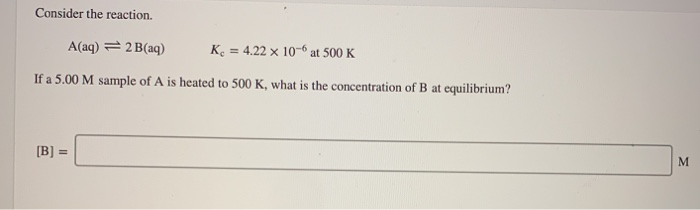Consider the reaction. A(aq) = 2 B(aq) K. = 4.22 x 10-6 at 500 K If a 5.00 M sample of A is heated to 500 K, what is the concentration of B at equilibrium? [B] = M

• ### Consider the reaction. A(aq) = 2 B(aq) Kc = 5.34 x 10-6 at 500 K If...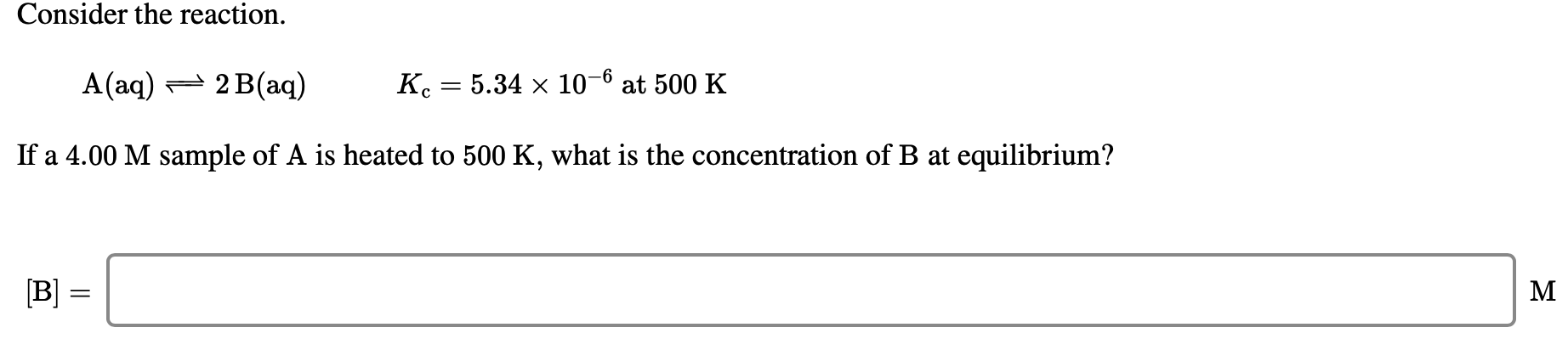Consider the reaction. A(aq) = 2 B(aq) Kc = 5.34 x 10-6 at 500 K If a 4.00 M sample of A is heated to 500 K, what is the concentration of B at equilibrium? [B] =

• ### Consider the reaction. A(aq) = 3 B(aq) K. = 9.88 x 10-6 at 500 K If...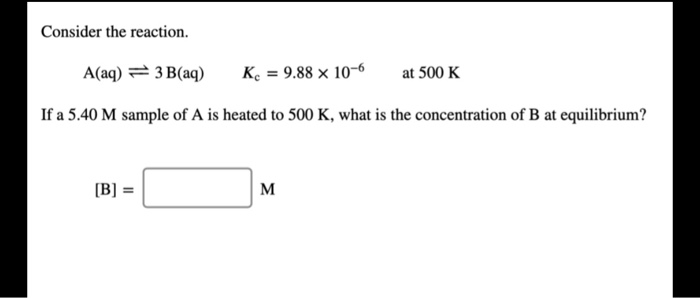Consider the reaction. A(aq) = 3 B(aq) K. = 9.88 x 10-6 at 500 K If a 5.40 M sample of A is heated to 500 K, what is the concentration of B at equilibrium?

• ### Consider the reaction. A(aq) = 3 B(aq) K= 6.40 x 10-6 at 500 K If a...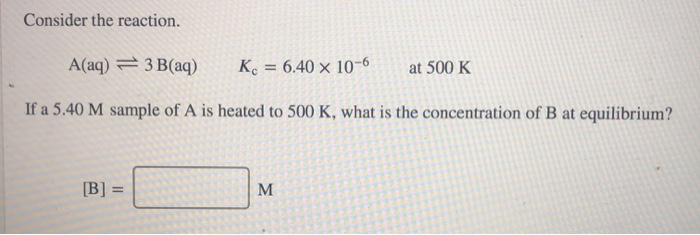Consider the reaction. A(aq) = 3 B(aq) K= 6.40 x 10-6 at 500 K If a 5.40 M sample of A is heated to 500 K, what is the concentration of B at equilibrium? B) =

• ### Consider the reaction. A(aq) = 3 B(aq) K. 2.00 x 10-6 at 500 K If a...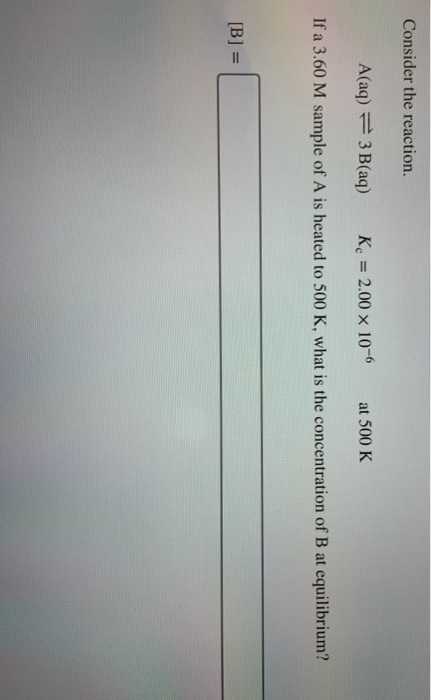Consider the reaction. A(aq) = 3 B(aq) K. 2.00 x 10-6 at 500 K If a 3.60 M sample of A is heated to 500 K, what is the concentration of B at equilibrium? [B] =

• ### Consider the reaction. A(aq) = 3 B(aq) K. = 6.30 x 10-6 at 500 K If...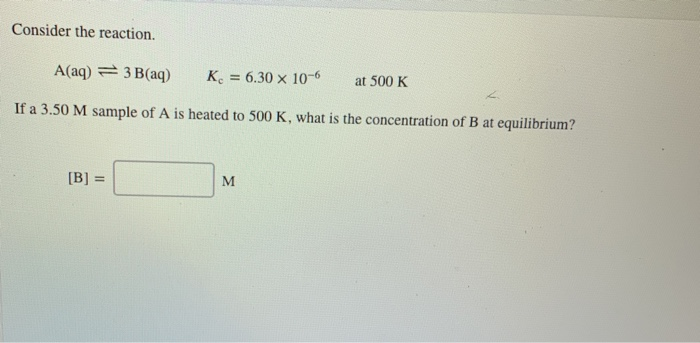Consider the reaction. A(aq) = 3 B(aq) K. = 6.30 x 10-6 at 500 K If a 3.50 M sample of A is heated to 500 K, what is the concentration of B at equilibrium? [B] = M

Free Homework App